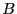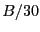Next: Example Up: Finding bounds on the Previous: Implementation   Contents

Global implementation

The above algorithms have been regrouped in two procedures, one for determining the bound on the positive roots, the other for the negative roots:

int Global_Positive_Bound_Interval(int Degree,VECTOR &Coeff,INTERVAL &Bound);
int Global_Negative_Bound_Interval(int Degree,VECTOR &Coeff,INTERVAL &Bound);
with:
• Degree: degree of the polynomial
• Coeff: the Degree+1 coefficients of the polynomial in increasing degree
• Bound: the bound on the positive or negative roots
For the positive root if Bound=[a,b] and b=0, then there is no positive root. Similarly for the negative root is a=0, then there is no negative root. The procedure returns 0 in case of failure which correspond to the failure of all the previous algorithms, 1 in case of success. Note that for Laguerre and Newton method the maximum number of iteration is defined by the global variable Max_Iter_Laguerre_Interval fixed by default to 1000. The step size is defined as:
• if a boundhave been previously determined, then the step size is fixed toexcept if the global variable Step_Laguerre_Interval has been defined to be a double not equal to 0, in which case this variable is used as the step size.
• if a bound as not been determined the step size is fixed to 1 except if the global variable
Step_Laguerre_Interval has been defined to be a double not equal to 0, in which case this variable is used as the step size.
• if the step if lower than the global variable Min_Step_Laguerre_Interval (which is 0.1 by default) the step is substituted by Min_Step_Laguerre_Interval
Similar procedure exist for interval polynomial:

int Global_Positive_Bound_Interval(int Degree,INTERVAL_VECTOR &Coeff,
INTERVAL &Lower,INTERVAL &Upper);
int Global_Negative_Bound_Interval(int Degree,INTERVAL_VECTOR &Coeff,
INTERVAL &Lower,INTERVAL &Upper);
where Lower is an interval on the lower bound: for positive roots and if Lower=[a,b] then the roots of any polynomial in the set is greater than a while some polynomial have root greater than b. Conversely if Upper=[a,b] the roots of any polynomial in the set is lower than b while some polynomial have root lower than a.

Both procedures for real roots have been regrouped in

void ALIAS_Find_Bound_Polynom(int Degree,
INTERVAL_VECTOR (* TheCoeff)(INTERVAL_VECTOR &),
INTERVAL_VECTOR &PALL,INTERVAL &Space, INTERVAL &Bound)
where
• PALL: the first component is the bound for the real roots the polynomial while the following are the value of the parameters for which the bounds will be obtained
• Space: a default bound for the real roots; may be used to indicate that we are looking only for bounds on the positive or negative real roots by specifying a positive or a negative lower bound
• Bound: bounds for the real roots

SubsectionsNext: Example Up: Finding bounds on the Previous: Implementation   Contents
Jean-Pierre Merlet 2012-12-20